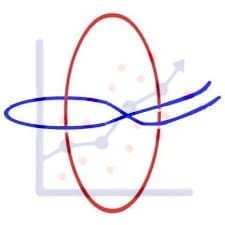Get inspired by the success stories of our students in IIT JAM MS, ISI  MStat, CMI MSc Data Science.  Learn More

# ISI MStat PSB 2005 Problem 1 | The Inductive MatrixThis is a very beautiful sample problem from ISI MStat PSB 2005 Problem 1. It is based on some basic properties of upper triangular matrix and diagonal matrix, only if you use them carefully. Give it a thought!

## Problem- ISI MStat PSB 2005 Problem 1

Let $A$ be a $n \times n$ upper triangular matrix such that $AA^T=A^TA$. Show that $A$ is a diagonal matrix.

### Prerequisites

Upper Triangular Matrix

Diagonal Matrix

Mathematical Induction

## Solution :

This is very beautiful problem, since it deals with some very beautiful aspects of matrix. Lets assume the matrix $A$ to be,

$A=\begin{bmatrix} a_{11} & \vec{{a_1}^T} \\ \vec{0_{n-1}}& A_{n-1} \end{bmatrix}$.

Here, $A_{n-1}$ is a partition matrix of $A$, which is also an upper triangular matrix of order $n-1$, and $\vec{0_{n-1}}$ is a null column vector of order $n-1$.

So, $AA^T= \begin{bmatrix} {a_{11}}^2+\vec{{a_1}^T}\vec{a_1} && \vec{{a_1}^T}{A_{n-1}}^T \\ A_{n-1}\vec{a_1}&& A_{n-1}{A_{n-1}}^T \end{bmatrix}$ .

Again, $A^TA= \begin{bmatrix} {a_{11}}^2&& a_{11}\vec{a_1} \\ a_{11}\vec{a_1}&& \vec{a_1}\vec{{a_1}^T}+{A_{n-1}}^TA_{n-1} \end{bmatrix}$.

Now lets assume that when a $n\times n$ upper triangular matrix $A$, holds $AA^T=A^TA$ , then $A$ is diagonal is true. Then equating the above elements, we have,

$\vec{{a_1}^T}\vec{a_1}=0 \Rightarrow \vec{a_1}=\vec{0}$ , and also $A_{n-1}{A_{n-1}}^T={A_{n-1}}^TA_{n-1}$.

Now observe that, if $A_{n-1}$ (which is actually an $n\times n$ upper triangular matrix), is a diagonal matrix then only $A$ will be also diagonal. So, its the same problem we are trying to prove !! So, just use induction get the proof done !!

## Food For Thought

What if I change the given relation as $AA^*=A^*A$, where $A^*$ is the conjugate matrix of $A$, rest of the conditions remains same. Can you investigate whether $A$ is a diagonal matrix or not ?

Keep thinking !!

## Subscribe to Cheenta at Youtube

This is a very beautiful sample problem from ISI MStat PSB 2005 Problem 1. It is based on some basic properties of upper triangular matrix and diagonal matrix, only if you use them carefully. Give it a thought!

## Problem- ISI MStat PSB 2005 Problem 1

Let $A$ be a $n \times n$ upper triangular matrix such that $AA^T=A^TA$. Show that $A$ is a diagonal matrix.

### Prerequisites

Upper Triangular Matrix

Diagonal Matrix

Mathematical Induction

## Solution :

This is very beautiful problem, since it deals with some very beautiful aspects of matrix. Lets assume the matrix $A$ to be,

$A=\begin{bmatrix} a_{11} & \vec{{a_1}^T} \\ \vec{0_{n-1}}& A_{n-1} \end{bmatrix}$.

Here, $A_{n-1}$ is a partition matrix of $A$, which is also an upper triangular matrix of order $n-1$, and $\vec{0_{n-1}}$ is a null column vector of order $n-1$.

So, $AA^T= \begin{bmatrix} {a_{11}}^2+\vec{{a_1}^T}\vec{a_1} && \vec{{a_1}^T}{A_{n-1}}^T \\ A_{n-1}\vec{a_1}&& A_{n-1}{A_{n-1}}^T \end{bmatrix}$ .

Again, $A^TA= \begin{bmatrix} {a_{11}}^2&& a_{11}\vec{a_1} \\ a_{11}\vec{a_1}&& \vec{a_1}\vec{{a_1}^T}+{A_{n-1}}^TA_{n-1} \end{bmatrix}$.

Now lets assume that when a $n\times n$ upper triangular matrix $A$, holds $AA^T=A^TA$ , then $A$ is diagonal is true. Then equating the above elements, we have,

$\vec{{a_1}^T}\vec{a_1}=0 \Rightarrow \vec{a_1}=\vec{0}$ , and also $A_{n-1}{A_{n-1}}^T={A_{n-1}}^TA_{n-1}$.

Now observe that, if $A_{n-1}$ (which is actually an $n\times n$ upper triangular matrix), is a diagonal matrix then only $A$ will be also diagonal. So, its the same problem we are trying to prove !! So, just use induction get the proof done !!

## Food For Thought

What if I change the given relation as $AA^*=A^*A$, where $A^*$ is the conjugate matrix of $A$, rest of the conditions remains same. Can you investigate whether $A$ is a diagonal matrix or not ?

Keep thinking !!

## Subscribe to Cheenta at Youtube

This site uses Akismet to reduce spam. Learn how your comment data is processed.

### Knowledge Partner